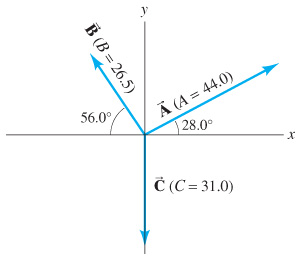Physics Practice Problems Vector Addition & Subtraction Practice Problems Solution: Given the vectors A and B shown in the figure, det...

⚠️Our tutors found the solution shown to be helpful for the problem you're searching for. We don't have the exact solution yet.

# Solution: Given the vectors A and B shown in the figure, determine B - A. Determine A - B without using your answer in part A.

###### Problem

Given the vectors A and B shown in the figure, determine B - A.#### Q. A 650-N force acts in a northwesterly direction.A second 650-N force must be exerted in what direction so that the resultant of the two forces points ...

Solved • Mon Oct 29 2018 19:00:32 GMT-0400 (EDT)

#### Q. A plumber steps out of his truck, walks 66 m east and 35 m south, and then takes an elevator 12 m into the subbasement of a building where a bad leak ...

Solved • Mon Oct 29 2018 19:00:25 GMT-0400 (EDT)